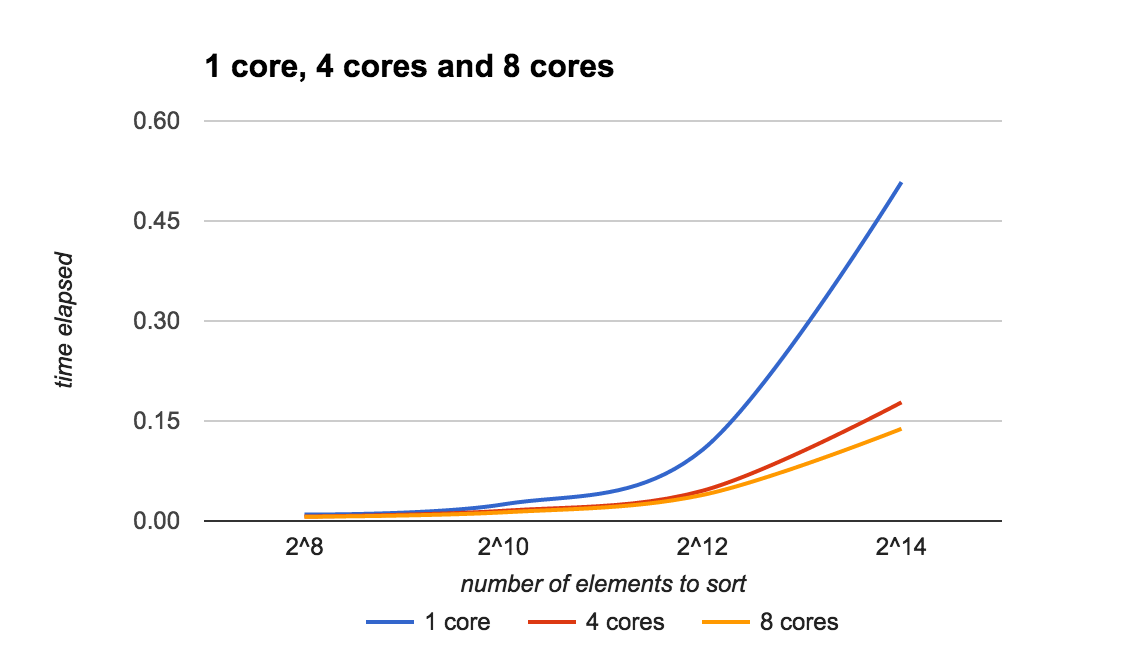# Bitonic Sort in Go

## 0. Context

This semester I’m enrolled in CSE6220: Into to HPC class, where Prof. Vuduc tries to make sure we have a lot of fun (and learn some HPC stuff along the way).

For the first assignment, we were expected to turn a simple program into equally simple program but one that can execute some of its instructions in parallel. The program is bitonic sorter written in C, and all we had to do was to inject a single OpenMP instruction. After running the resultant code on Tech’s DeepThought cluster, I got puzzled with the question: how hard will it be to implement the very same bitonic sorter in Go?

Turned out, it was trivial to do!

So, this post is a quick summary of the work done. Please note that implemented sorter is primitive at best:

• it runs on a single machine (with multiple cores), no distribution to separate nodes
• it sorts only integers (easy to fix, but requires some typing..nobody mention generics here!)

## 1. Prerequisites

If you wonder what Bitonic Sort is, you definitely wonder what Sorting Networks are all about.

Here are some links to get you up to speed in no time:

For our purposes it is enough to understand that a sorting network is a special kind of sorting algorithm, where the sequence of comparisons is not data-dependent.

Sounds like a good case for parallel execution, right?

## 2. Implementation

You know what is really nice about Bitonic Sort? Not only it can perform really well, it is really easy to implement.

Here is the full code:

package bitonic

const (
SORT_ASC  bool = true
SORT_DESC bool = false
)

var lst []int

func Sort(input []int, dir bool) {
lst = input
sentinel := make(chan int)
go bitonicSort(0, len(lst), dir, sentinel)
<-sentinel
}

func bitonicSort(lo int, n int, dir bool, sentinel chan int) {
if n > 1 {
m := n / 2
c1 := make(chan int)
c2 := make(chan int)
go bitonicSort(lo, m, SORT_ASC, c1)
go bitonicSort(lo+m, m, SORT_DESC, c2)
<-c1
<-c2
bitonicMerge(lo, n, dir, sentinel)
} else {
sentinel <- 1
}
}

func bitonicMerge(lo int, n int, dir bool, sentinel chan int) {
if n > 1 {
m := n / 2
for i := lo; i < lo+m; i++ {
compareAndSwap(i, i+m, dir)
}
c1 := make(chan int)
c2 := make(chan int)
go bitonicMerge(lo, m, dir, c1)
go bitonicMerge(lo+m, m, dir, c2)
<-c1
<-c2
}

sentinel <- 1
}

func compareAndSwap(i int, j int, dir bool) {
if dir == (lst[i] > lst[j]) {
lst[i], lst[j] = lst[j], lst[i]
}
}


## 3. Analysis (kind of)

You can do your own experiments by forking farazdagi/bitonic repo.

Here is what I did:

• tried to run on a single core, on 4 cores, and on 8 cores
• every test got three runs, average time was taken
• the very same code was run every time i.e. even in case of a single core run, I used exactly the same concurrent program.

Here is a what I got:Notes:

• as you see elapsed time to sort $2^{14}$ items on a single processor quickly goes up. Our parallel execution on multiple processors can handle the load easily.
• $2^{20} = 1.048.576$ and it takes ~$14$ seconds to sort those 1M records on 8-core machine. Pretty impressive.
• on second figure, when we compare 4 and 8 cores, difference is not that striking. I guess our parallel execution becomes dominated by memory accesses (sorting is in shared memory).

## 4. Summary and Take-Aways

1. Go is truly amazing language and I will definitely experiment with its concurrency model more (as we proceed with CSE6220). So, you should probably expect more posts similar to this one.
2. If you got interested in Bitonic sort, here is a list of awesomeness:

Don’t forget to fork the repo. You can also follow me on Twitter.

Have a nice day and happy hacking!